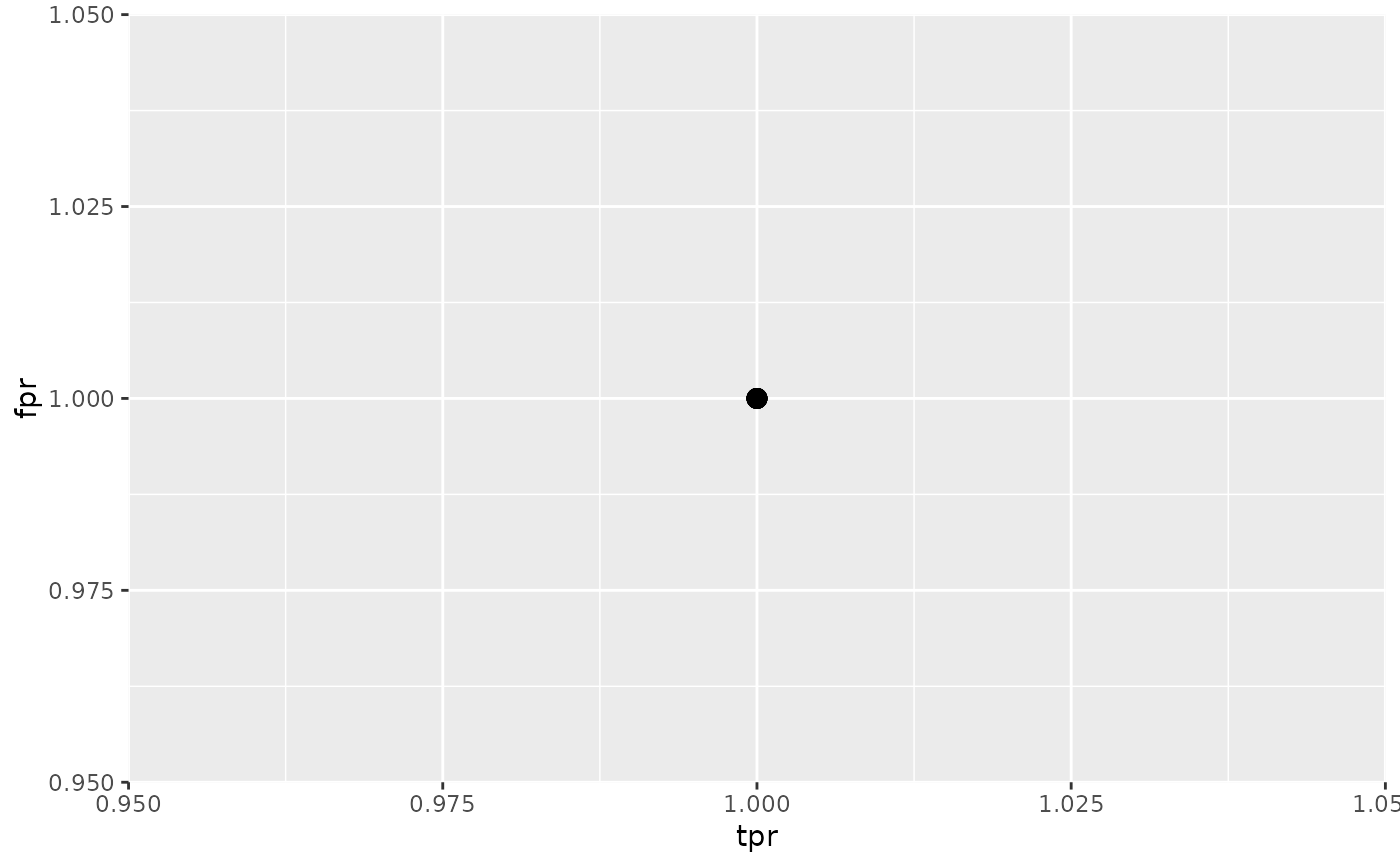Optimizes the hyperparameters of a learner in a multi-criteria fashion. Allows for different optimization methods, such as grid search, evolutionary strategies, etc. You can select such an algorithm (and its settings) by passing a corresponding control object. For a complete list of implemented algorithms look at TuneMultiCritControl.

tuneParamsMultiCrit(
learner,
resampling,
measures,
par.set,
control,
show.info = getMlrOption("show.info"),
resample.fun = resample
)

## Arguments

learner (Learner | character(1)) The learner. If you pass a string the learner will be created via makeLearner. (Task) The task. (ResampleInstance | ResampleDesc) Resampling strategy to evaluate points in hyperparameter space. If you pass a description, it is instantiated once at the beginning by default, so all points are evaluated on the same training/test sets. If you want to change that behavior, look at TuneMultiCritControl. [list of Measure) Performance measures to optimize simultaneously. (ParamHelpers::ParamSet) Collection of parameters and their constraints for optimization. Dependent parameters with a requires field must use quote and not expression to define it. (TuneMultiCritControl) Control object for search method. Also selects the optimization algorithm for tuning. (logical(1)) Print verbose output on console? Default is set via configureMlr. (closure) The function to use for resampling. Defaults to resample and should take the same arguments as, and return the same result type as, resample.

## Value

Other tune_multicrit: TuneMultiCritControl, plotTuneMultiCritResult()

## Examples

# \donttest{
# multi-criteria optimization of (tpr, fpr) with NGSA-II
lrn = makeLearner("classif.ksvm")
rdesc = makeResampleDesc("Holdout")
ps = makeParamSet(
makeNumericParam("C", lower = -12, upper = 12, trafo = function(x) 2^x),
makeNumericParam("sigma", lower = -12, upper = 12, trafo = function(x) 2^x)
)
ctrl = makeTuneMultiCritControlNSGA2(popsize = 4L, generations = 1L)
res = tuneParamsMultiCrit(lrn, sonar.task, rdesc, par.set = ps,
measures = list(tpr, fpr), control = ctrl)
#> [Tune] Started tuning learner classif.ksvm for parameter set:#>          Type len Def    Constr Req Tunable Trafo
#> C     numeric   -   - -12 to 12   -    TRUE     Y
#> sigma numeric   -   - -12 to 12   -    TRUE     Y#> With control class: TuneMultiCritControlNSGA2#> Imputation value: -0Imputation value: 1#> [Tune-x] 1: C=0.0036; sigma=0.207#> [Tune-y] 1: tpr.test.mean=1.0000000,fpr.test.mean=1.0000000; time: 0.0 min#> [Tune-x] 2: C=0.072; sigma=0.244#> [Tune-y] 2: tpr.test.mean=1.0000000,fpr.test.mean=1.0000000; time: 0.0 min#> [Tune-x] 3: C=0.0384; sigma=2.73#> [Tune-y] 3: tpr.test.mean=1.0000000,fpr.test.mean=1.0000000; time: 0.0 min#> [Tune-x] 4: C=0.00326; sigma=1.99e+03#> [Tune-y] 4: tpr.test.mean=1.0000000,fpr.test.mean=1.0000000; time: 0.0 min#> [Tune-x] 5: C=0.338; sigma=0.207#> [Tune-y] 5: tpr.test.mean=1.0000000,fpr.test.mean=1.0000000; time: 0.0 min#> [Tune-x] 6: C=0.00335; sigma=0.244#> [Tune-y] 6: tpr.test.mean=1.0000000,fpr.test.mean=1.0000000; time: 0.0 min#> [Tune-x] 7: C=0.0882; sigma=0.145#> [Tune-y] 7: tpr.test.mean=1.0000000,fpr.test.mean=1.0000000; time: 0.0 min#> [Tune-x] 8: C=0.00267; sigma=1.99e+03#> [Tune-y] 8: tpr.test.mean=1.0000000,fpr.test.mean=1.0000000; time: 0.0 min#> [Tune] Result: Points on front : 8plotTuneMultiCritResult(res, path = TRUE)# }